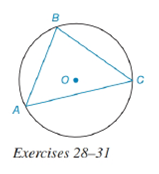Chapter 6.4, Problem 29EElementary Geometry For College St...

7th Edition
Alexander + 2 others
ISBN: 9781337614085

Solutions

Chapter
SectionElementary Geometry For College St...

7th Edition
Alexander + 2 others
ISBN: 9781337614085
Textbook Problem

Given circle O with m B C ⌢ = 120 ° , and m A C ⌢ = 130 ° .a) Which angle of triangle A B C is smallest?b) Which side of triangle A B C is nearest point O ?To determine

(a)

To find:

The smallest angle of triangle ABC.

Explanation

Given:

Circle O with mBC=120°, and mAC=130°. The figure given below

Property Used:

The measure of an inscribed angle of a circle is half the measure of its intercepted arc. Consider the figure below, let the inscribed angle be 2

Then m2=12mHJ and generally HKKJ.

Calculation:

Since, the measure of an inscribed angle of a circle is half the measure of its intercepted arc and from the given figure

To determine

(b)

To find:

The side of triangle ABC that is nearest point O.

Still sussing out bartleby?

Check out a sample textbook solution.

See a sample solution

The Solution to Your Study Problems

Bartleby provides explanations to thousands of textbook problems written by our experts, many with advanced degrees!

Get Started

Solve the equations in Exercises 112 for x (mentally, if possible). 4x5=8

Finite Mathematics and Applied Calculus (MindTap Course List)

13. .

Mathematical Applications for the Management, Life, and Social Sciences

Define the terms population and sample, and explain the role of each in a research study.

Essentials of Statistics for The Behavioral Sciences (MindTap Course List)

Find f. f(x) = 2 + 12x 12x2, f(0) = 4, f(0) = 12

Single Variable Calculus: Early Transcendentals

True or False: converges.

Study Guide for Stewart's Multivariable Calculus, 8th